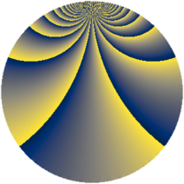# Properties

 Label 1148.2.bnLevel $1148$ Weight $2$ Character orbit 1148.bn Rep. character $\chi_{1148}(169,\cdot)$ Character field $\Q(\zeta_{20})$ Dimension $176$ Sturm bound $336$

# Learn more about

## Defining parameters

 Level: $$N$$ $$=$$ $$1148 = 2^{2} \cdot 7 \cdot 41$$ Weight: $$k$$ $$=$$ $$2$$ Character orbit: $$[\chi]$$ $$=$$ 1148.bn (of order $$20$$ and degree $$8$$) Character conductor: $$\operatorname{cond}(\chi)$$ $$=$$ $$41$$ Character field: $$\Q(\zeta_{20})$$ Sturm bound: $$336$$

## Dimensions

The following table gives the dimensions of various subspaces of $$M_{2}(1148, [\chi])$$.

Total New Old
Modular forms 1392 176 1216
Cusp forms 1296 176 1120
Eisenstein series 96 0 96

## Trace form

 $$176q + 4q^{3} + O(q^{10})$$ $$176q + 4q^{3} - 4q^{13} - 32q^{15} + 8q^{17} + 56q^{19} - 12q^{23} + 68q^{25} - 20q^{27} + 20q^{31} - 4q^{35} - 16q^{37} + 80q^{39} + 16q^{41} + 48q^{45} + 8q^{47} - 8q^{51} + 72q^{55} + 8q^{63} + 4q^{65} - 12q^{67} - 68q^{69} - 68q^{71} + 56q^{75} - 140q^{79} - 264q^{81} + 104q^{83} - 96q^{85} - 160q^{87} + 12q^{89} - 16q^{93} - 184q^{95} - 100q^{97} - 128q^{99} + O(q^{100})$$

## Decomposition of $$S_{2}^{\mathrm{new}}(1148, [\chi])$$ into newform subspaces

The newforms in this space have not yet been added to the LMFDB.

## Decomposition of $$S_{2}^{\mathrm{old}}(1148, [\chi])$$ into lower level spaces

$$S_{2}^{\mathrm{old}}(1148, [\chi]) \cong$$ $$S_{2}^{\mathrm{new}}(41, [\chi])$$$$^{\oplus 6}$$$$\oplus$$$$S_{2}^{\mathrm{new}}(82, [\chi])$$$$^{\oplus 4}$$$$\oplus$$$$S_{2}^{\mathrm{new}}(164, [\chi])$$$$^{\oplus 2}$$$$\oplus$$$$S_{2}^{\mathrm{new}}(287, [\chi])$$$$^{\oplus 3}$$$$\oplus$$$$S_{2}^{\mathrm{new}}(574, [\chi])$$$$^{\oplus 2}$$